## Structure elements and their energy contribution

In this section, we classify the structure elements defining a secondary structure and describe their energy contribution.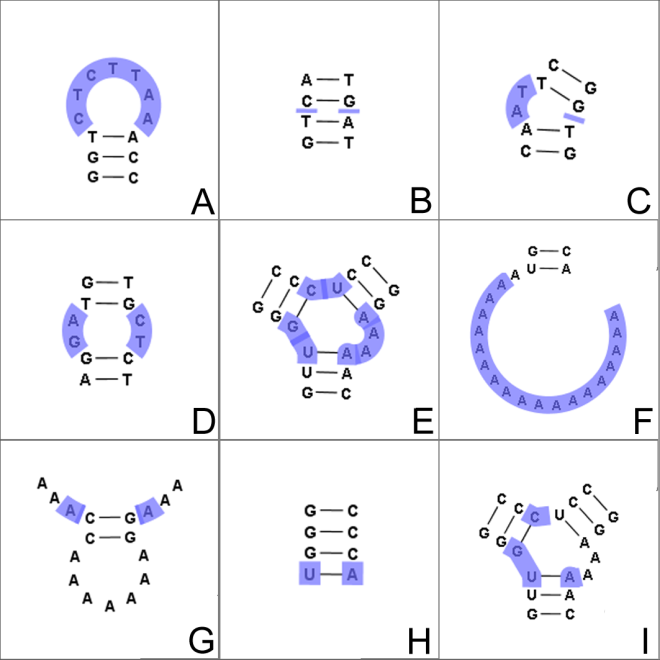Figure 24.29: The different structure elements of RNA secondary structures predicted with the free energy minimization algorithm in CLC Genomics Workbench. See text for a detailed description.

#### Nested structure elements

The structure elements involving nested base pairs can be classified by a given base pair and the other base pairs that are nested and accessible from this pair. For a more elaborate description we refer the reader to [Sankoff et al., 1983] and [Zuker and Sankoff, 1984].

If the nucleotides with position number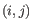form a base pair and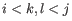, then we say that the base pair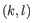is accessible fromif there is no intermediate base pair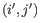such that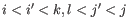. This means thatis nested within the pair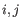and there is no other base pair in between.

Using the number of accessible pase pairs, we can define the following distinct structure elements:

1. Hairpin loop (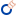). A base pair with 0 other accessible base pairs forms a hairpin loop. The energy contribution of a hairpin is determined by the length of the unpaired (loop) region and the two bases adjacent to the closing base pair which is termed a terminal mismatch (see figure 24.29A).
2. A base pair with 1 accessible base pair can give rise to three distinct structure elements:
• Stacking of base pairs (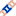). A stacking of two consecutive pairs occur if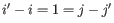. Only canonical base pairs (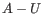or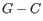or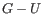) are allowed (see figure 24.29B). The energy contribution is determined by the type and order of the two base pairs.
• Bulge (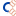). A bulge loop occurs if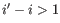or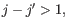but not both. This means that the two base pairs enclose an unpaired region of length 0 on one side and an unpaired region of length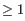on the other side (see figure 24.29C). The energy contribution of a bulge is determined by the length of the unpaired (loop) region and the two closing base pairs.
• Interior loop (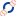).An interior loop occurs if bothand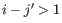This means that the two base pairs enclose an unpaired region of lengthon both sides (see figure 24.29D). The energy contribution of an interior loop is determined by the length of the unpaired (loop) region and the four unpaired bases adjacent to the opening- and the closing base pair.
3. Multi loop opened (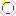). A base pair with more than two accessible base pairs gives rise to a multi loop, a loop from which three or more stems are opened (see figure 24.29E). The energy contribution of a multi loop depends on the number of Stems opened in multi-loop (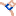) that protrude from the loop.

#### Other structure elements

• A collection of single stranded bases not accessible from any base pair is called an exterior (or external) loop (see figure 24.29F). These regions do not contribute to the total free energy.

• Dangling nucleotide (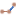). A dangling nucleotide is a single stranded nucleotide that forms a stacking interaction with an adjacent base pair. A dangling nucleotide can be a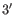or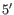-dangling nucleotide depending on the orientation (see figure 24.29G). The energy contribution is determined by the single stranded nucleotide, its orientation and on the adjacent base pair.

• Non-GC terminating stem (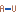). If a base pair other than a G-C pair is found at the end of a stem, an energy penalty is assigned (see figure 24.29H).

• Coaxial interaction (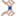). Coaxial stacking is a favorable interaction of two stems where the base pairs at the ends can form a stacking interaction. This can occur between stems in a multi loop and between the stems of two different sequential structures. Coaxial stacking can occur between stems with no intervening nucleotides (adjacent stems) and between stems with one intervening nucleotide from each strand (see figure 24.29I). The energy contribution is determined by the adjacent base pairs and the intervening nucleotides.

#### Experimental constraints

A number of techniques are available for probing RNA structures. These techniques can determine individual components of an existing structure such as the existence of a given base pair. It is possible to add such experimental constraints to the secondary structure prediction based on free energy minimization (see figure 24.30) and it has been shown that this can dramatically increase the fidelity of the secondary structure prediction [Mathews and Turner, 2006].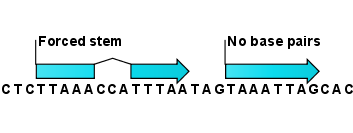Figure 24.30: Known structural features can be added as constraints to the secondary structure prediction algorithm in CLC Genomics Workbench.